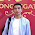## Friday, March 7, 2014

### Trigonometric Function

Every forms can be defined with coordinates, dimensions, and angles. And these components can be found each other with formulas.

As it is known, trigonometric function is the most useful formula in this case.

See the graph below.

Values of sine and cosine are always between -1 and +1. It means any angle can be found with the value between 0 and 1(plus or minus).

There is a similar one in Revit.

The location of a point on a line can be defined with the value between 0 and 1 in Revit.

So it can be said that a point can be located on a line with angle by using trigonometric function, and vise versa.

Here is an example I have made.(This is the first uploaded video in my channel)

(Yeah, I love LEGO)

And here is the how to video.

I made it just for fun. but the concept can be used in real architecture projects.
(you can download the family above here, except the figure)

Enjoy ;-)

1.2.